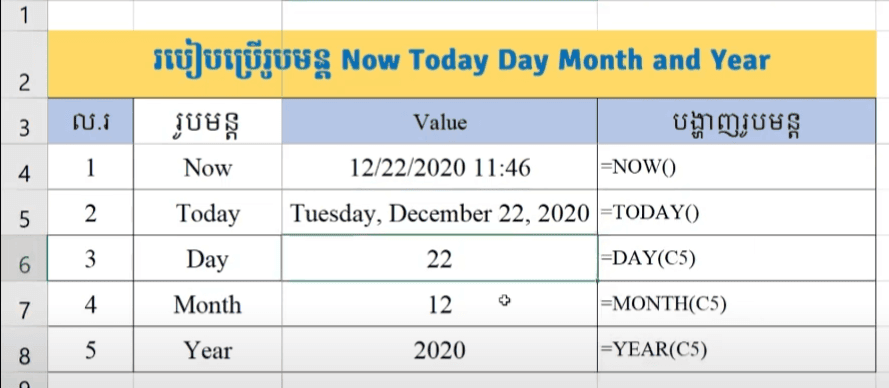# 39. Date Formulas in Excel (Now, Today, Day, Month and Year)

Date Formulas in Excel (Now, Today, Day, Month and Year)
In this Excel tutorial show you the different Now() and Today() formula and also how to get Day, Month, Year from date too.NOW Formula: return current date and time
=NOW()

TODAY Formula: return current date only
=TODAY()

DAY Formula: return number of day from date
=DAY(serial_number)
◾ serial_number: Required, is your date.

Month Formula: return Month from date
=Month(serial_number)
◾ serial_number: Required, is your date.

Year Formula: return year from date
=Year(serial_number)
◾ serial_number: Required, is your date.